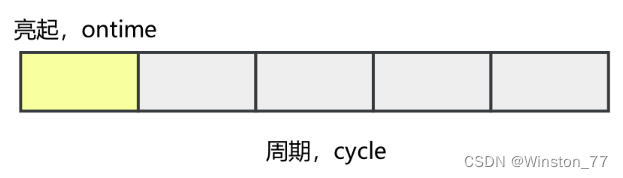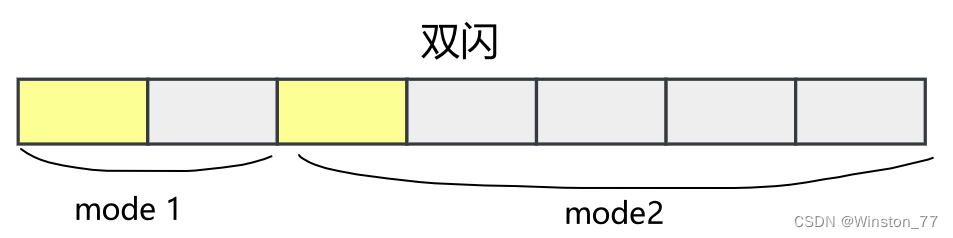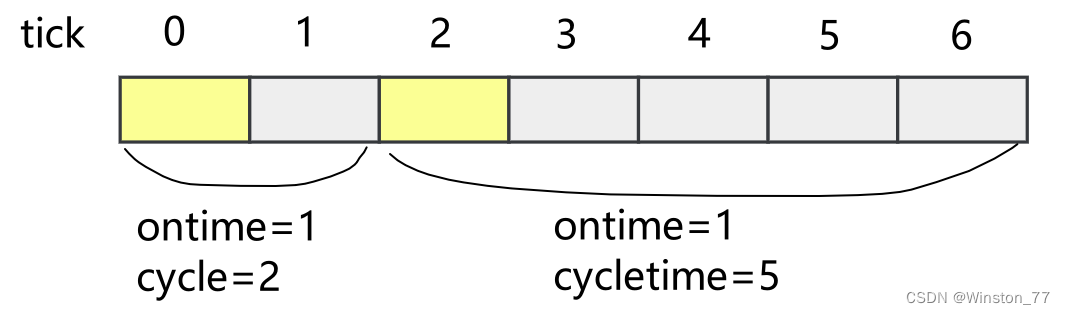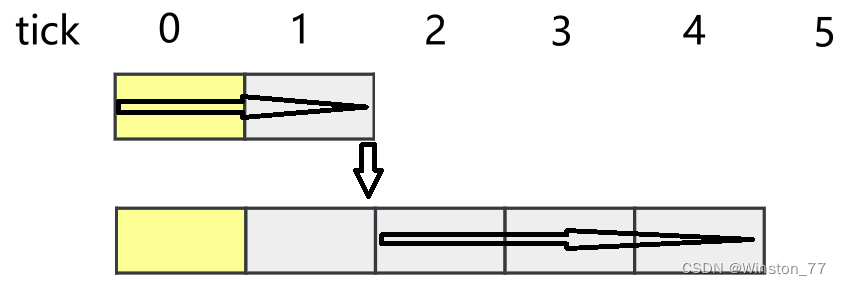## 单片机中的通用LED驱动

chatgpt/2023/9/27 6:55:10

## 前言

• 方便的增加不同闪烁模式
• 可以切换闪烁模式
• 增加LED数目不会有太多的改动
• 方便移植，要有良好的硬件对接接口

PS：本文中的程序源码只做演示，可运行的代码文末有链接

## 数据结构分析

``````typedef struct{void (*init)(void);	//初始化EDvoid (*on)(void);	//打开LEDvoid (*off)(void);	//关闭LED
}led_t;
``````

LED闪烁是亮灭的交替，我们可以关注其中的两个参数，

• LED亮起时长，标记为ontime
• LED闪烁周期，标记为cycle将这两个参数抽象为led_mode_t结构体
``````typedef struct {uint16_t cycle;		//LED闪烁周期uint16_t ontime;	//LED亮起的时长
}led_mode_t;
``````

``````typedef struct{void (*init)(void);	//初始化EDvoid (*on)(void);	//打开LEDvoid (*off)(void);	//关闭LEDled_mode_t *mode;		//LED闪烁模式具体的数据uint8_t mode_count;		//LED闪烁模式个数uint8_t mode_current;	//当前闪烁模式编码
}led_t;
``````

OK，LED结构体初步搭建完毕，接下来假设我们有4颗LED，每一颗闪烁的时间参数如下

• 快闪：200ms亮起，200ms熄灭，周期400ms
• 慢闪：500ms亮起，500ms熄灭，周期1000ms
• 单闪：100ms亮起，900ms熄灭，周期1000ms
• 双闪：30ms亮起， 70ms熄灭，30ms亮起， 870ms熄灭，周期1000ms## 程序框架搭建

``````typedef struct {uint16_t cycle;		//LED闪烁周期uint16_t ontime;	//LED亮起的时长
}led_mode_t;typedef struct{void (*init)(void);	//初始化EDvoid (*on)(void);	//打开LEDvoid (*off)(void);	//关闭LEDled_mode_t *mode;		//LED闪烁模式具体的数据uint8_t mode_count;		//LED闪烁模式个数uint8_t mode_current;	//当前闪烁模式编码
}led_t;led_t led_array;void led0_init(void){}
void led1_init(void){}
void led2_init(void){}
void led3_init(void){}
void led0_on(void){}
void led1_on(void){}
void led2_on(void){}
void led3_on(void){}
void led0_off(void){}
void led1_off(void){}
void led2_off(void){}
void led3_off(void){}void bsp_led_init(void)
{//初始化函数指针led_array.on = led0_on;led_array.off = led0_off;led_array.init = led0_init;led_array.mode_count = 1;led_array.on = led1_on;led_array.off = led1_off;led_array.init = led1_init;led_array.mode_count = 1;	led_array.on = led2_on;led_array.off = led2_off;led_array.init = led2_init;led_array.mode_count = 1;led_array.on = led3_on;led_array.off = led3_off;led_array.init = led3_init;led_array.mode_count = 2;for(uint8_t i = 0; i < sizeof(led_array)/led_array; i++){led_array[i].mode = malloc(sizeof(led_mode_t) * led_array[i].mode_count);memset(led_array[i].mode, 0, sizeof(led_mode_t) * led_array[i].mode_count);}//初始化mode时间参数led_array.mode.ontime = 200;	led_array.mode.cycle 	= 400;led_array.mode.ontime = 500;led_array.mode.cycle 	= 1000;led_array.mode.ontime = 100;led_array.mode.cycle 	= 1000;led_array.mode.ontime = 30;led_array.mode.cycle 	= 100;led_array.mode.ontime = 30;led_array.mode.cycle 	= 900;
}
``````

• 每一个led都有init，on，off函数
• 初始化函数指针
• 初始化mode时间参数

``````#define INIT_PTR(__index, __onptr, __offptr, __initptr, __modecount)	\led_array[__index].on = __onptr;									\led_array[__index].off = __offptr;									\led_array[__index].init = __initptr;								\led_array[__index].mode_count = __modecount;						#define INIT_MODE(__index, __mode, __ontime, __cycle)	\led_array[__index].mode[__mode].ontime = __ontime;	\led_array[__index].mode[__mode].cycle 	= __cycle;
``````

``````void bsp_led_init(void)
{INIT_PTR(0, led0_on, led0_off, led0_init, 1);INIT_PTR(1, led1_on, led1_off, led1_init, 1);INIT_PTR(2, led2_on, led2_off, led2_init, 1);INIT_PTR(3, led3_on, led3_off, led3_init, 2);for(uint8_t i = 0; i < sizeof(led_array)/led_array; i++){led_array[i].mode = malloc(sizeof(led_mode_t) * led_array[i].mode_count);memset(led_array[i].mode, 0, sizeof(led_mode_t) * led_array[i].mode_count);}INIT_MODE(0, 0, 200, 400);INIT_MODE(1, 0, 500, 1000);INIT_MODE(2, 0, 100, 100);INIT_MODE(3, 0, 30, 100);INIT_MODE(3, 1, 30, 900);
}
``````

OK，我们已经设定了各个LED的闪烁模式，对接了初始化，亮起，熄灭的函数，是时候让他跑起来了。

``````static void bsp_led_tick(void){
#define i_CYCLE_LENGTH  (led_array[i].mode[led_array[i].mode_current].cycle)
#define i_ON_TIME (led_array[i].mode[led_array[i].mode_current].ontime)static uint64_t tick;for(uint8_t i = 0; i <  i < sizeof(led_array)/led_array; i++){if(tick % i_CYCLE_LENGTH < i_ON_TIME)led_array[i].on();elseled_array[i].off();}tick++;
}
``````

## 问题修复

LED关闭可以认为是一种模式。其中ontime为0，周期为任意值（0除外）

``````	···for(uint8_t i = 0; i < sizeof(led_array)/led_array; i++){//增加关闭模式，此后mode就被占用了，用户定义的闪烁模式要从mode开始led_array[i].mode = malloc(sizeof(led_mode_t) * (led_array[i].mode_count)+1);memset(led_array[i].mode, 0, sizeof(led_mode_t) *  (led_array[i].mode_count)+1t);}INIT_MODE(0, 1, 200, 400);INIT_MODE(1, 1, 500, 1000);INIT_MODE(2, 1, 100, 100);INIT_MODE(3, 1, 30, 100);INIT_MODE(3, 2, 30, 900);···
``````
``````static void bsp_led_tick(void){······//cycletime == 0， LED不再闪烁if(i_CYCLE_LENGTH == 0){if( (lled_array[i]..state == 1)){led_array[i].state = 0;led_array[i].off();}continue;}else{if(tick % i_CYCLE_LENGTH < i_ON_TIME){if( (led_array[i].state == 0)){//如果之前是关闭的，那么开启led_array[i].state = 1;led_array[i].on();}}else{if( led_array.led[i].state == 1){//如果之前是开启的，那么关闭led_array[i].state = 0;led_array[i].off();}}}tick++;
}
``````

``````//周期回调函数
if(i_CYCLE_LENGTH-1 == (tick % i_CYCLE_LENGTH))
{led_array[i].cycle_func();
}
``````

cycle_func是led_t中增加的新成员，作为周期结束的回调函数。使用前需要初始化指向led3_cyclefun，在该函数中做模式切换

``````static void led3_cyclefun()
{if(led_array.mode_current== 1)led_array.mode_current = 2;else if(led_array.mode_current== 2)led_array.mode_current = 1;
}
``````## 优化

``````typedef struct{led_t led[LED_COUNT];void (*on)(void *parameter);	//打开LEDvoid (*off)(void *parameter);	//关闭LEDvoid (* cycle_func)(void *parameter);	//cycle结束的回调函数
}led_array_t;
``````

``````static void bsp_led_on(void *parameter)
{led_t *p = (led_t *)parameter;switch(p-led_array.led){case 0:DRV_DIO_ChannelOutSet(DRV_DIO_ID_PIO_9);break;case 1:DRV_DIO_ChannelOutSet(DRV_DIO_ID_PIO_25);break;case 2:DRV_DIO_ChannelOutSet(DRV_DIO_ID_PIO_14);break;case 3:DRV_DIO_ChannelOutSet(DRV_DIO_ID_PIO_15);break;default:break;};
}
``````

OK，内容到此结束了，这样看来写好一个LED并不容易。

### 以科技创新引领短交通行业发展，九号公司重磅新品亮相巴塞罗那MWC

2月27日&#xff0c;以“时不我待(VELOCITY) - 明日科技&#xff0c;将至已至”为主题的2023世界移动通信大会&#xff08;Mobile World Congress&#xff0c;以下简称MWC&#xff09;在西班牙巴塞罗那举办&#xff0c;全球创新短交通领军企业九号公司参加了大会。现场&#xf…

### RBAC三级树状菜单实现（从前端到后端）未完待续

1、表格设计 RBAC 2、前端路由 根据不同的用户id显示不同的菜单。 根据路由 3、多级菜单 展示所有权限&#xff0c;并且根据当前用户id展示它所属的角色的所有菜单。 前端树状展示 思路&#xff1a; 后端&#xff1a;传给前端map&#xff0c;map里1个是所有菜单&am…